### Production And Costs Class 12th Introductory Microeconomics CBSE Solution

##### Question 1.Explain the concept of a production function.Answer:Production function explains the relationship between factor inputs and output, which means the relation between factors of production, their productivity, and final outcome. It may also be defined as the technological relationship between inputs and outputs.According to Prof. Watson"Production function is the relation between a firm's physical production (output) and the material factors of production (inputs).”Production may be defined as the conversion of physical inputs into physical output. As output depends upon input so we can say output is a function of inputs.There is a direct relationship between the number of inputs and the production of output. The production function is nothing other than a tool used to show this relationship.The production function is given as -Q = f (L,K,D...)Where –Q stands for - Output of the firmf stands for functionL, K, D stands for the physical quantity of Labour (L), Capital (K) and Land (D) used.Suppose we say that a firm uses two inputs - capital and labour, then the production function will indicate the physical relationship between these two inputs and output of the firm. It will be represented asQ = f (L,K)Where –Q stands for - Output of the firmf stands for functionL, K stands for the physical quantity of labour and capital used.Question 2.What is the total product of an input?Answer:Total product means the total volume of goods produced during the specified period of time. The total product can be increased only by increasing the quantity of variable input employed in production.For example, if a firm is producing shirts using labour and capital then as the quantity of labour will increase the total product will also increase but only up to a certain level that is up to the maximum capacity. Suppose the capacity of a firm is to keep 100 machines on which 100 workers can be employed then even if we increase the manpower to 150, it will not increase the total production rather it will reduce the capacity of labour.Let's go through the following product schedule to understand it –Now, see that with every increasing unit of labour the total product is increasing but the total product is constant when 5 men and 6 men are employed after that it is decreasing even when input is increasing. This means the maximum capacity of firm is to produce 170 units.Question 3.What is the average product of an input?Answer:Average product means dividing the total product by total number of units of variable factors, it is also known as per unit product of a variable factor.The mathematical expression for Average Product (AP) is –AP = Total Product/Units of Variable FactorLet's go through the following product schedule to understand it –The average product can never be zero.Question 4.What is the marginal product of an input?Answer:Marginal product is the rate at which total product increases or we can define the marginal product as the increase in the total product with every additional unit of the variable factor.For Example – If a firm produces 10 pieces of shirt by employing 1 labour and 15 pieces of shirt by employing 2 labours, then the marginal product will be 5.The mathematical expression for Marginal Product (MP) is –MPn= TPn – TPn-1Where,MPn – Marginal Product at ‘n’ units of variable factorTPn – Total Product at ‘n’ units of variable factorTPn-1 – Total Product at ‘n-1’ units of variable factorLet's go through the following product schedule to understand it –Now, see that with every increasing unit of labour the marginal product is increasing initially but when the total product is constant the marginal product is zero and then after it is negative. This means once the maximum capacity of firm is achieved then the marginal product becomes negative for every additional level of input.Question 5.Explain the relationship between the marginal products and the total product of an input.Answer:The relation between marginal product and total product is described as below –a) When Total Product increases at an increasing rate Marginal Product increases.b) When Total Product increases at a decreasing rate Marginal Product decreases but it is positive.c) When Total Product is maximum and constant Marginal Product becomes zero.d) When Total Product falls Marginal Product becomes negativeLet's go through the following product schedule to understand it –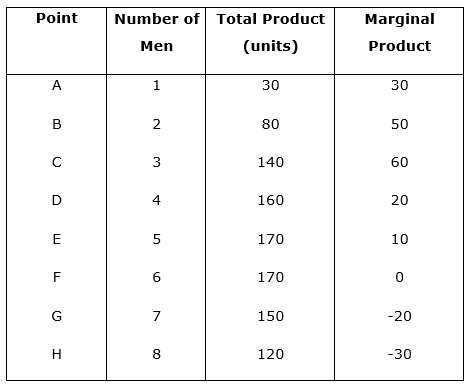• From point A – B & B – C, The Total Product is increasing at an increasing rate so the Marginal Product is also increasing.• From point C – D & D – E, The Total Product is increasing at a decreasing rate so the Marginal Product is decreasing.• From point E – F, The Total Product is constant so the Marginal Product is zero.• From point F – G & G – H, The Total Product is decreasing so the Marginal Product is negative.The following graph will make it easier to understand –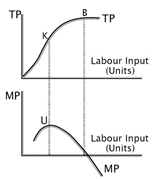Question 6.Explain the concepts of the short run and the long run.Answer:Short run may be defined as a period in which some factors are fixed and some are variable. During short run, it is not possible to increase all the factors of production but only the variable factors can be increased.The scale of production remains constant in short run. In short run, the output can be increased or decreased only by employing more or less of the variable factors, i.e labour.It is assumed that in short run the firm doesn't have sufficient time to increase the fixed factors and so the output is dependent only upon the level of the variable factor or we can say the output level can vary only because of the variable factor.Algebraically, short run production function is expressed as –Q = f(L, K)Where –Q – OutputL- LabourK – Constant units of CapitalIn long run all factors are variable that is all the factors can be changed to increase the output. In long run the scale of production can be changed. In long run a firm can change all its inputs so the output can be increased or decreased by employing more or less of any of the inputs.It is assumed that in long run the firm have sufficient time to increase the factors of input and so all the factors are variable. In long run “Returns to Scale” phenomenon is in operation.Algebraically, long run production function is expressed as –Q = f(L, K)Where –Q – OutputL- LabourK – CapitalBoth L & K are variableQuestion 7.What is the law of diminishing marginal product?Answer:The law of diminishing marginal product states that if we keep increasing the employment of an input assuming that other inputs are fixed the marginal product will increase only up to a particular level and then will start falling. Law of diminishing returns suggests that the stage where the return to inputs starts diminishing is the ultimate stage.In the initial stages as we increase the quantity of variable factors the total output will increase at an increasing rate and the marginal product will also increase.In the next stage as we increase the quantity of variable factors the total output will increase at a decreasing rate but the marginal product will increase.But once the total output is constant, that is there is no change in the total output with any change in the variable factor, the marginal product will be zero.When the total output decreases with increase in variable factor the marginal product will be negative.Let’s understand it with help of following product schedule and the graph –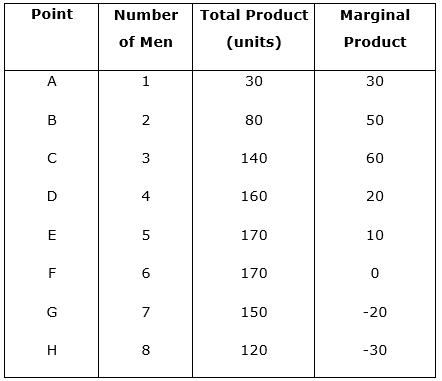Now, we will represent this data on graph –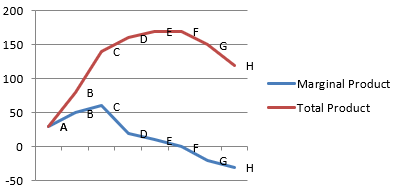Question 8.What is the law of variable proportions?Answer:Law of variable proportion explains the relation between the proportion of fixed and variable inputs on one hand and output on the other. When the firm increases its output by employing more of the units of the variable factor it changes the ratio between fixed and variable factors. The law speaks about three stages of production1st Stage - It goes from origin to the point where the average output is maximum. When a firm increases the output by increasing the quantity of variable factors in proportion to fixed factors it moves towards optimum combination factors of production at this stage the marginal input increases that is the total output increases at an increasing rate it is the stage where the law of increasing returns operates. The marginal output begins to fall at this stage and the law of diminishing distance is introduced.2nd stage - It starts from the point where the average output is maximum and goes to the point where the marginal output is zero. Once the optimum combination of fixed inputs and variable inputs is attained after that if the firm increases the quantity of variable output the per unit output of variable input that is average input will fall. In this stage, the total output rises but at a diminishing rate. This is a crucial stage for a firm because in this stage the firm determines its level of actual production.3rd stage - It covers the range over which the marginal output is negative. It is also known as the stage of negative returns. In this stage, the total output begins to fall. Thus the total output, average output, and marginal output all fall. No producer operates at this stage even if he can procure the variable factors for zero price.Let’s understand it with help of following table and graph –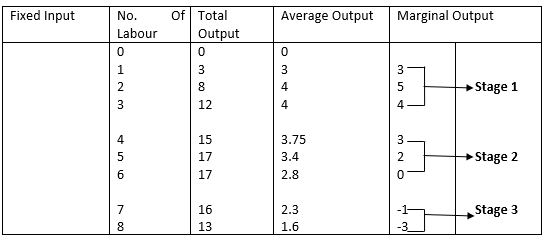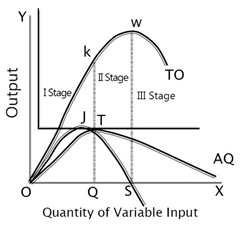Question 9.When does a production function satisfy constant returns to scale?Answer:Constant returns to scale means when increase in total output is proportional to increase in the quantities of inputs i.e there is equal increase in output and input.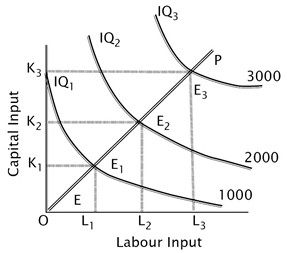Here, IQ1, IQ2 and IQ3 represent the total output of 1000 units, 2000 units and 3000 units respectively.As we can see EE1 = E1E2 = E2E3, so it is constant returns to scale.Therefore, constant returns to scale operate when total output increases exactly in the same proportion as an increase in the quantity of factor inputs.Question 10.When does a production function satisfy increasing returns to scale?Answer:When the total output increases in a larger proportion than the proportionate increase in the factor input, it is called increasing returns to scale.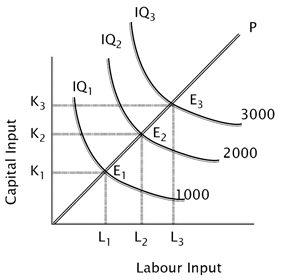Here, IQ1, IQ2 and IQ3 represent the total output of 1000 units, 2000 units and 3000 units respectively.As we can see EE1 > E1E2 > E2E3, so it is increasing returns to scale.Question 11.When does a production function satisfy decreasing returns to scale?Answer:Diminishing or decreasing returns to scale where the increase in output is more than proportionate increase in quantities of factor inputs it is called diminishing returns to scale.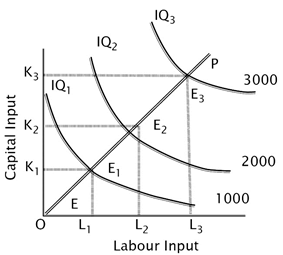Here, IQ1, IQ2 and IQ3 represent the total output of 1000 units, 2000 units and 3000 units respectively.As we can see EE1 < E1E2 < E2E3, so it is decreasing returns to scale.Question 12.Briefly explain the concept of the cost function.Answer:Cost function expresses the relationship between cost and its determinants. There are several factors that influence the cost which includes size of plant, price of input, level of output, technology, etc when their relationship is established with cost it is called cost function.The cost function can be formulated for short run as well as for long run but these two are interrelated. The cost function can be linear or nonlinear depending upon the nature and behaviour of cost.The cost function depicts the least cost combination of inputs associated with different level of inputs. Therefore, it depicts the output-cost relationship.Question 13.What are the total fixed cost, total variable cost and total cost of a firm? How are they related?Answer:a) Total fixed cost refers to the cost which remains constant irrespective to the level of output, the total fixed cost will never change. For example, suppose Mr X hired a shop at a dent of rupees 500 per month for selling grocery, during first month he keeps a shop closed even then he will have to pay the rent so rent is fixed cost.Let’s understand it with help of following cost schedule and graphCost ScheduleGraph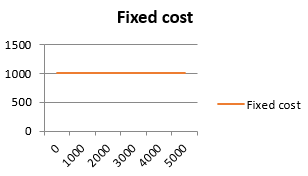b) Total variable cost is the cost of the variable inputs employed by the firm which is directly proportional to the level of change in the output. It becomes zero when the level of output is zero, increases with output, decreases with output.Let’s understand it with help of following cost schedule and graphCost Schedule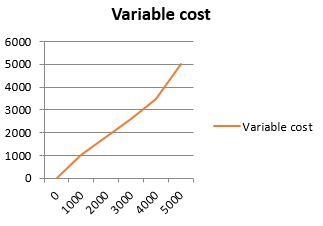c) Total cost is the sum of total fixed cost and total variable cost. At zero level of output total cost will be just the fixed cost.Let’s understand it with help of following cost schedule and graphCost ScheduleGraph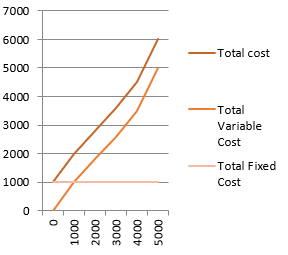Question 14.What are the average fixed cost, average variable cost and average cost of a firm? How are they related?Answer:Average fixed cost is per unit cost on fixed factors, it decreases with increase in output.Average fixed cost = Total Fixed Cost / No. of Units ProducedCost ScheduleGraph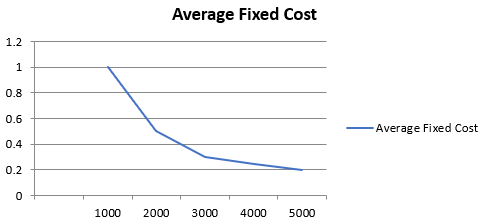Average variable cost is per unit cost of variable factors it remains constant.Average variable cost = Total Variable Cost / No. of Units ProducedCost ScheduleGraph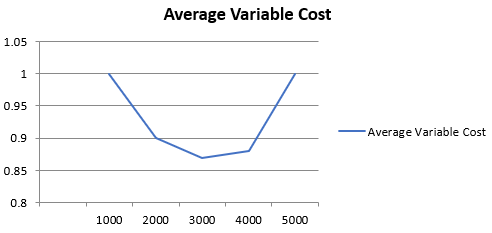Average total cost means total cost per unit of output it is a u shaped curve like average variable cost. The average fixed cost is represented by a rectangular hyperbolaAverage total cost = Total Cost / No. of Units ProducedCost ScheduleGraph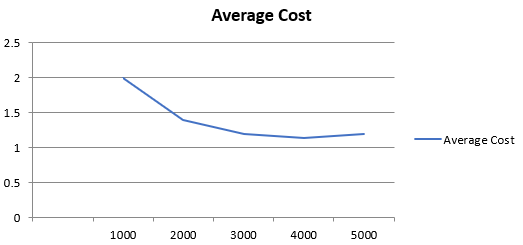Relationship between Average Fixed Cost, Average Variable Cost, and Average Cost -• Average fixed cost curve is rectangular hyperbola.• It falls continuously to the right but will never touch the x-axis because average fixed cost can never be zero.• Average variable cost and average total cost or average cost curve is U shaped.• Average cost curve lies above average variable cost curve and as output increases the vertical difference between two curves will decrease but they will never intersect.Question 15.Can there be some fixed cost in the long run? If not, why?Answer:In long run all the cost are variable cost because the firm has sufficient time to change the factors of input. So there is no fixed cost in long run therefore in long run total cost will be equal to total variable cost.Question 16.What does the average fixed cost curve look like? Why does it look so?Answer:Average fixed cost curve looks like a rectangular hyperbola. The average fixed cost is total fixed cost divided by the output. As we know that total fixed cost remains same so as the output will increase the average fixed cost will decrease.At low level of output, that is something near 0 the average fixed cost will be very high as compared to the average fixed cost at maximum level of output. However it never become zero because fixed cost is never 0, so it is like a rectangular hyperbola and never intersects the x axis.The following schedule and graph will help to understand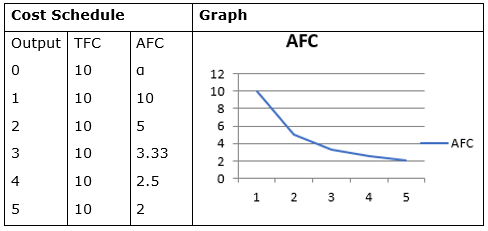TFC – Total Fixed CostAFC – Average Fixed Cost = Total Fixed Cost / outputQuestion 17.What do the short run marginal cost, average variable cost and short run average cost curves look like?Answer:The short run marginal cost (SMC), average variable cost (AVC) and short run average cost (SAC) curves are all u shaped.The reason behind it is the law of variable proportion, which states that in initial stages of production in short run the average and marginal cost fall.Subsequently with constant returns to labour the cost curve become constant and reach their minimum point, which represents the optimum combination of capital and Labour. Beyond this with every additional unit of labour will increase the cost and as the marginal product of labour starts falling the cost curve starts rising due to decrease in returns to labour.Let's understand it with help of graph –Cost Schedule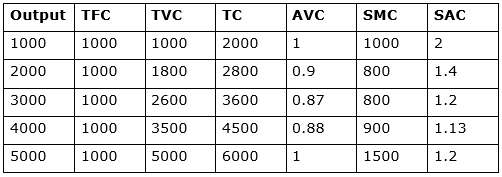GRAPH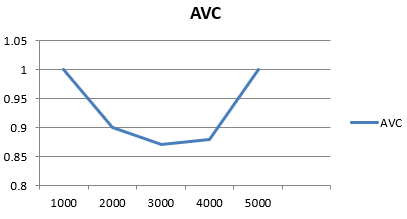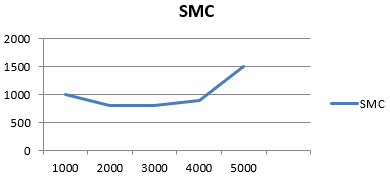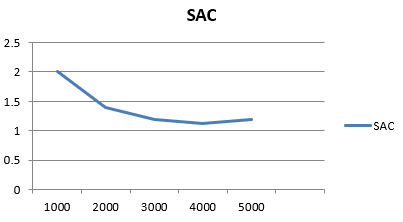Question 18.Why does the SMC curve cut the AVC curve at the minimum point of the AVC curve?Answer:The SMC curve always intersects the AVC curve at its minimum, because at the minimum point of AVC the SMC is below AVC.Both SMC and AVC fall but SMC Falls at a faster rate than AVC. Say the minimum point is K where AVC is equal to SMC beyond this point the AVC and SMC both rises but SMC Rises at a faster rate and is above AVC therefore the only point where SMC and AVC are equal is where is SMC intersects AC that is the minimum point KLet's understand it with help of graph –Cost ScheduleGRAPH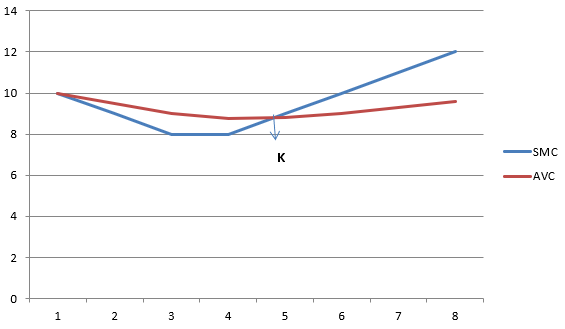Question 19.At which point does the SMC curve cut the SAC curve? Give reason in support of your answer.Answer:Short run marginal cost (SMC) curve cuts the short run average cost (SAC) curve from below at the minimum point of SAC curve, where SAC is constant and at its minimum point.The reason behind it is -• When SMC and SAC fall, the SMC falls at a lower rate than SAC• When SMC and SAC rise, the SMC rises at a greater rate than SACLet's understand it with help of graph –Cost ScheduleGRAPH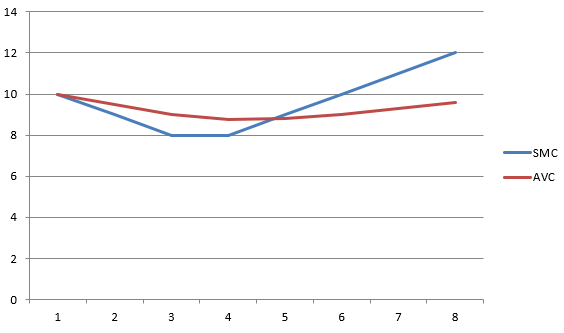Question 20.Why is the short run marginal cost curve ‘U’-shaped?Answer:The short run marginal cost curve is ‘U’ shaped because initially the marginal cost falls but ultimately it rises. It is based upon the law of variable proportions.Let's understand it with help of graph –Cost ScheduleGRAPH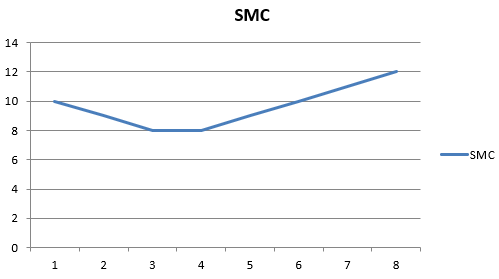Question 21.What do the long run marginal cost and the average cost curves look like?Answer:The long run marginal cost (LMC) curve and long range average cost (LAC) curves are U shaped curves but flatter than short run U shape, because of the law of returns to scale.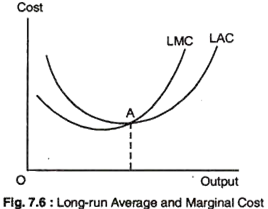The important factors determining the shape of LAC and LMC are that whether there is increasing, constant, or decreasing returns to scale.• At constant returns to scale AC will be same for all levels of output• At increasing returns to scale the average cost with fall will fall with every unit of output• At decreasing returns to scale the average cost will increase with every unit of outputQuestion 22.The following table gives the total product schedule of labour. Find the corresponding average product and marginal product schedules of labour.Answer:MP – Marginal Product = TPN+1 - TPNAP – Average Product = TP / LQuestion 23.The following table gives the average product schedule of labour. Find the total product and marginal product schedules. It is given that the total product is zero at zero level of labour employment.Answer:Given – Total product of labour is zero at zero level of employmentThis means that labour is variable factor.MP – Marginal Product = TPN+1 - TPNTP – Total Product = AP X LQuestion 24.The following table gives the marginal product schedule of labour. It is also given that total product of labour is zero at zero level of employment. Calculate the total and average product schedules of labour.Answer:Given – Total product of labour is zero at zero level of employmentThis means that labour is variable factor.TP – Total Product = MPN+1 + MPNAP – Average Product = TP / LQuestion 25.The following table shows the total cost schedule of a firm. What is the total fixed cost schedule of this firm? Calculate the TVC, AFC, AVC, SAC and SMC schedules of the firm.Answer:The total cost given for 0 output is Rs 10, it means the fixed cost is Rs 10SAC = TC / QTFC = 10TVC = TC – TFCAVC = TVC / QAFC = TFC / QSMC = TVCN+1 - TVCNQuestion 26.The following table gives the total cost schedule of a firm. It is also given that the average fixed cost at 4 units of output is Rs 5. Find the TVC, TFC, AVC, AFC, SAC and SMC schedules of the firm for the corresponding values of output.Answer: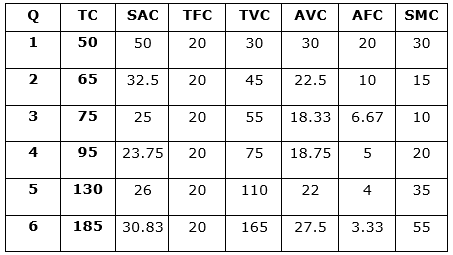Given – Average fixed cost at 4 units of output is Rs 5Therefore, Total Fixed Cost for 4 units will be Rs 20As we know that the FC remains constant so it will be Rs 20 for all levels of outputSAC = TC / QTFC = 20TVC = TC – TFCAVC = TVC / QAFC = TFC / QSMC = TVCN+1 - TVCNQuestion 27.A firm’s SMC schedule is shown in the following table. The total fixed cost of the firm is Rs 100. Find the TVC, TC, AVC and SAC schedules of the firm.Answer: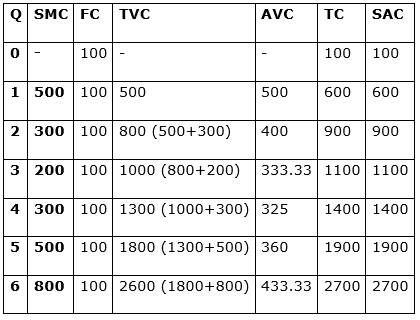TVC = SMCN + SMCN+1AVC = TVC / QTC = FC + TVCSAC = TC / QQuestion 28.Let the production function of a firm beQ = 5L1/2K1/2Find out the maximum possible output that the firm can produce with 100 units of L and 100 units of K.Answer:The production function of a firm is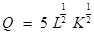Where,Q – OutputL – LabourK – CapitalGiven,L = 100K = 100Therefore, Q = 5(100) 1/2 + 2 (100)1/2Q = 5 X 10 X 10Q = 500Question 29.Let the production function of a firm beQ = 2L2K2Find out the maximum possible output that the firm can produce with 5 units of L and 2 units of K. What is the maximum possible output that the firm can produce with zero unit of L and 10 units of K?Answer:The production function of a firm is –Q = 2L2K2Where,Q – OutputL – LabourK – CapitalGiven,L = 5K = 2Therefore, Q = 2 (5)2(2)2Q = 2 X 25 X 4Q = 200When,L = 0K = 10Therefore, Q = 2 (0)2(10)2Q = 2 X 0 X 100Q = 0Question 30.Find out the maximum possible output for a firm with zero unit of L and 10 units of K when its production function is Q = 5L + 2KAnswer:The production function is given by –Q = 5L + 2KWhere,Q – OutputL – LabourK – CapitalGiven,L = 0K = 10Therefore, Q = 5(0) + 2 (10)Q = 0 + 20Q = 20

PDF FILE TO YOUR EMAIL IMMEDIATELY PURCHASE NOTES & PAPER SOLUTION. @ Rs. 50/- each (GST extra)

HINDI ENTIRE PAPER SOLUTION

MARATHI PAPER SOLUTION

SSC MATHS I PAPER SOLUTION

SSC MATHS II PAPER SOLUTION

SSC SCIENCE I PAPER SOLUTION

SSC SCIENCE II PAPER SOLUTION

SSC ENGLISH PAPER SOLUTION

SSC & HSC ENGLISH WRITING SKILL

HSC ACCOUNTS NOTES

HSC OCM NOTES

HSC ECONOMICS NOTES

HSC SECRETARIAL PRACTICE NOTES

# 2019 Board Paper Solution

HSC ENGLISH SET A 2019 21st February, 2019

HSC ENGLISH SET B 2019 21st February, 2019

HSC ENGLISH SET C 2019 21st February, 2019

HSC ENGLISH SET D 2019 21st February, 2019

SECRETARIAL PRACTICE (S.P) 2019 25th February, 2019

HSC XII PHYSICS 2019 25th February, 2019

CHEMISTRY XII HSC SOLUTION 27th, February, 2019

OCM PAPER SOLUTION 2019 27th, February, 2019

HSC MATHS PAPER SOLUTION COMMERCE, 2nd March, 2019

HSC MATHS PAPER SOLUTION SCIENCE 2nd, March, 2019

SSC ENGLISH STD 10 5TH MARCH, 2019.

HSC XII ACCOUNTS 2019 6th March, 2019

HSC XII BIOLOGY 2019 6TH March, 2019

HSC XII ECONOMICS 9Th March 2019

SSC Maths I March 2019 Solution 10th Standard11th, March, 2019

SSC MATHS II MARCH 2019 SOLUTION 10TH STD.13th March, 2019

SSC SCIENCE I MARCH 2019 SOLUTION 10TH STD. 15th March, 2019.

SSC SCIENCE II MARCH 2019 SOLUTION 10TH STD. 18th March, 2019.

SSC SOCIAL SCIENCE I MARCH 2019 SOLUTION20th March, 2019

SSC SOCIAL SCIENCE II MARCH 2019 SOLUTION, 22nd March, 2019

XII CBSE - BOARD - MARCH - 2019 ENGLISH - QP + SOLUTIONS, 2nd March, 2019

# HSCMaharashtraBoardPapers2020

(Std 12th English Medium)

HSC ECONOMICS MARCH 2020

HSC OCM MARCH 2020

HSC ACCOUNTS MARCH 2020

HSC S.P. MARCH 2020

HSC ENGLISH MARCH 2020

HSC HINDI MARCH 2020

HSC MARATHI MARCH 2020

HSC MATHS MARCH 2020

# SSCMaharashtraBoardPapers2020

(Std 10th English Medium)

English MARCH 2020

HindI MARCH 2020

Hindi (Composite) MARCH 2020

Marathi MARCH 2020

Mathematics (Paper 1) MARCH 2020

Mathematics (Paper 2) MARCH 2020

Sanskrit MARCH 2020

Sanskrit (Composite) MARCH 2020

Science (Paper 1) MARCH 2020

Science (Paper 2)

Geography Model Set 1 2020-2021

MUST REMEMBER THINGS on the day of Exam

Are you prepared? for English Grammar in Board Exam.

Paper Presentation In Board Exam

How to Score Good Marks in SSC Board Exams

Tips To Score More Than 90% Marks In 12th Board Exam

How to write English exams?

How to prepare for board exam when less time is left

How to memorise what you learn for board exam

No. 1 Simple Hack, you can try out, in preparing for Board Exam

How to Study for CBSE Class 10 Board Exams Subject Wise Tips?

JEE Main 2020 Registration Process – Exam Pattern & Important Dates

NEET UG 2020 Registration Process Exam Pattern & Important Dates

How can One Prepare for two Competitive Exams at the same time?

8 Proven Tips to Handle Anxiety before Exams!